# Prime Recycling ζ(s) (syntax)

Warning

This page is referring to child section which source is inherited by the prime spin with partitions listed below. Please return always to the Home Page to track the hierarchy.

As shown in The Primes Platform this cycle goes to the prime 13 then reinjected through index 13:9 . So it forms The Scheme-139 through the infinitely primes cycling. This scheme will be implemented by taking the following as the syntax algorithm: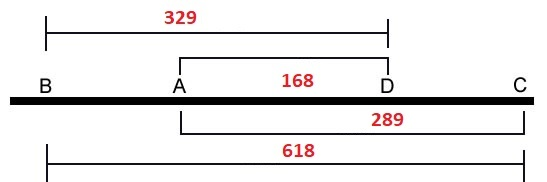``````Direction:
- The initial of 168 & 329 brings the 102 as 100+2 to π(π(10000))-1=200 or 100 x 2.
- Then the 289 lets this 100x2 to 100² so it brings 100 to 10000 by the power of 2.
- At the last it will be separated by the scheme of 168 to 102 goes back 100 and 2.

Conclution:
- All of the other primes than 2 is 1 less than the number n times the number of 2.
- Those Mersenne primes is generated as 1 less than the power n of the number of 2.
- Thus they will conseqently be carried out by the same scheme of this number of 2.
``````

This 23 units will form Scheme-23 as two (2) long strands which is known as doble helix Here we call them as Scheme-23 (71) and Scheme-23 (68). These strands are originated by the three (3) layers of True Prime Pairs.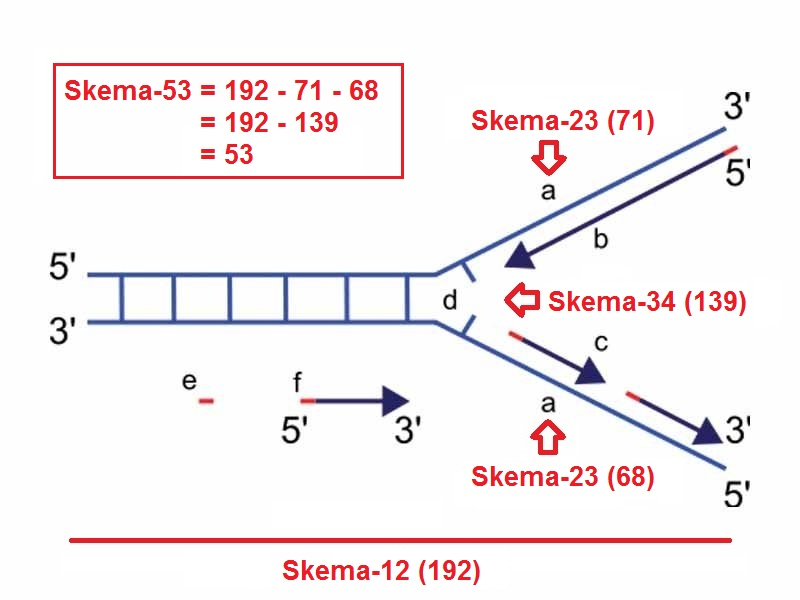The Ulam spiral or prime spiral is a graphical depiction of the set of prime numbers, devised by mathematician Stanisław Ulam in 1963 and popularized in Martin Gardner's Mathematical Games column in Scientific American a short time later.

Both Ulam and Gardner noted that the existence of such prominent lines is not unexpected, as lines in the spiral correspond to quadratic polynomials, and certain such polynomials, such as Euler's prime-generating polynomial x²-x+41, are believed to produce a high density of prime numbers. Nevertheless, the Ulam spiral is connected with major unsolved problems in number theory such as Landau's problems (Wikipedia).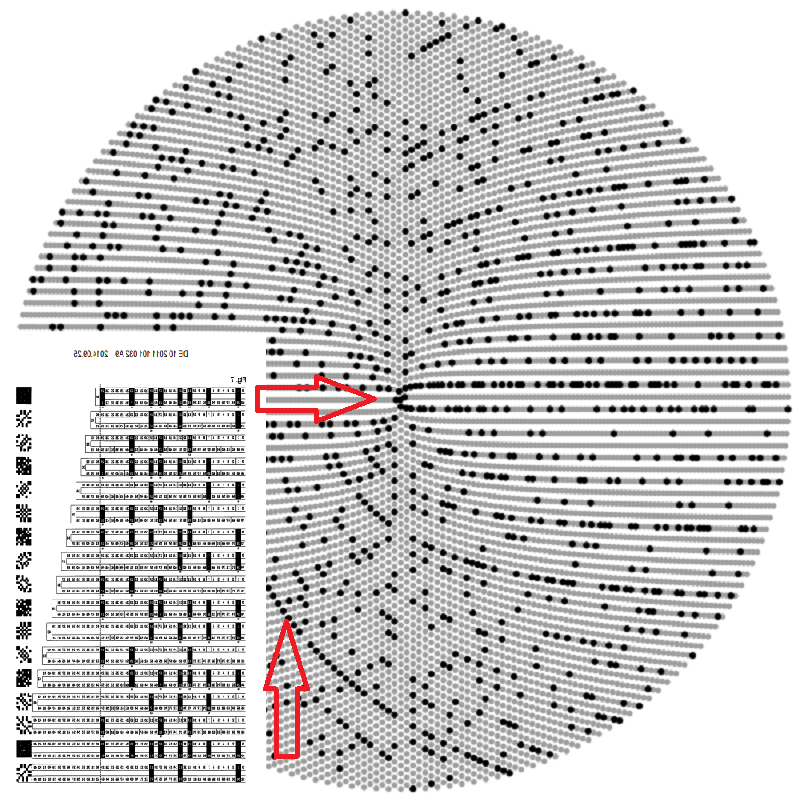This pattern is raised up per six (6) cycles on the 19, 43 and 71. Since the members are limited to the sum of 43+71=114 so here the bilateral way of 19 that originated by the (Δ1) is clearly the one that controls the scheme.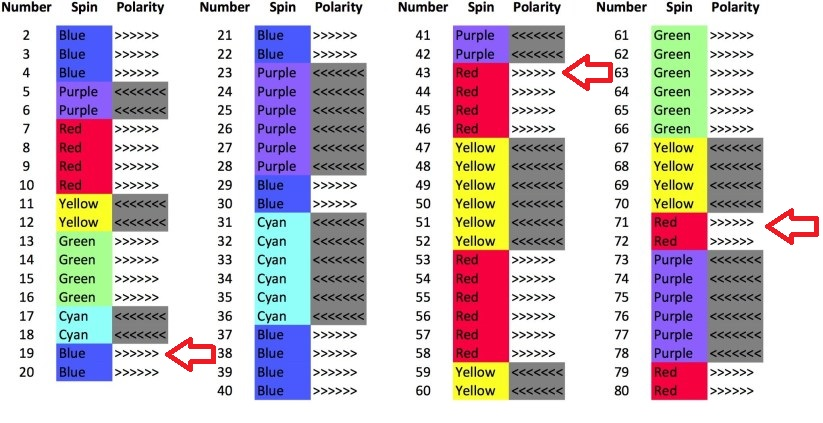This property would tend the ballancing scheme of MEC30 so it will let 30-18=12 pairing with another 12 of 24 spins prime hexagon. The 24 goes to the center of True Prime Pairs ny prime pair 13 and 11 and let the crancks of 2,3,5,7 inside the 10 ranks.

Current versions of string theory require 10 dimensions total, while an even more hypothetical über-string theory known as M-theory requires 11. But when we look around the universe, we only ever see the usual three spatial dimensions plus the dimension of time (Expert Voices).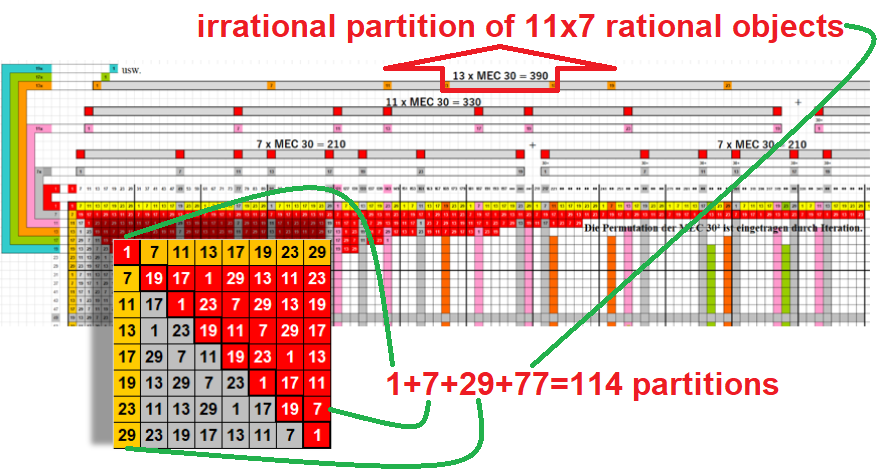By the prime hexagon this number 114 located on 6th row vs 19th column whereas 114th prime = 619 = 618+1. Since DNA Recombination is happen when two (2) chromosomes involve then they start the position as below:

``````                                                       |               by the 1st chromosome   |  by the 2nd
+----+----+----+----+----+----+----+----+----+----+----+----+----+----+----+----+----+----+----+----+----+
Δ18 |  - | 30 | 36 |  - |  - |  - |  - |  - |  - |  - |  - |  - | -  |102 |   -|  - |  - |  - | 30 | 36 |  - |
=====+====+====+====+====+====+====+====+====+====+====+====+====+====+====+====+====+====+====+====+====+====+
1  |  2 |  3 |  4 |  5 |  6 |  7 |  8 |  9 | 10 | 11 | 12 | 13 | 14 | 15 |  16|  17| 18 | 19 | 20 | 21 | 22 |
-----+----+----+----+----+----+----+----+----+----+----+----+----+----+----+----+----+----+----+----+----+----+
|       Δ    Δ                |                     Φ12     |       Δ                   Δ |
113  150                                   ≜114-25          557                619 = 1+618
``````

See that both will take a total of 19 + 17 = 36 collums, means it always back to 4 root position. By this cycling finally then 900 - 618 - 168 = 114 primes will take their natural segregation and stand as the last standing grammar.